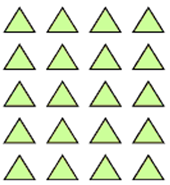Mathematics
Easy

Question

# 5 × 4 = _______.## 15    10    25    20Hint:

## The correct answer is: 20

### In the question there is an array using which we have to find the product of the expression for the given array.Number of columns in the given array= 4Number of rows in the given array= 5Expression of the given array= 5 × 4The product of the expression for the given array= 5 × 4 =20So, the product of the expression for the given array is 20.Therefore, the correct option is d, i.e., 20.

For simple multiplication we have to remember the multiplication table of one-digit numbers and for two-digit number we can find it using the multiplication table of one-digit numbers. Here, we have to find the product of one digit number.

### Related Questions to study#### With Turito Foundation.#### Get an Expert Advice From Turito.